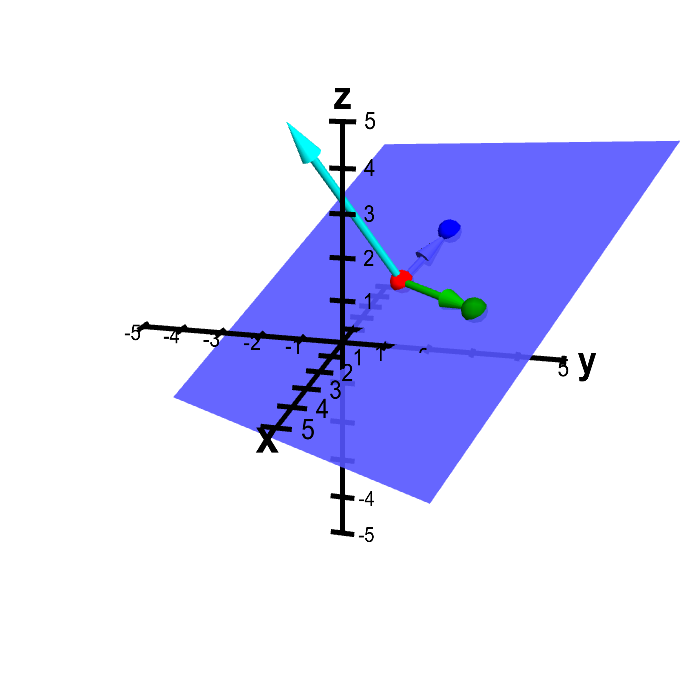# Math Insight

### Applet: Plane determined from three pointsThe plane is determined by the points $\color{red}{P}$ (in red), $\color{green}{Q}$ (in green), and $\color{blue}{R}$ (in blue), which you can move by dragging with the mouse. The vectors from $\color{red}{P}$ to both $\color{green}{Q}$ and $\color{blue}{R}$ are drawn in the corresponding colors. The normal vector (in cyan) is the cross product of the green and blue vectors.